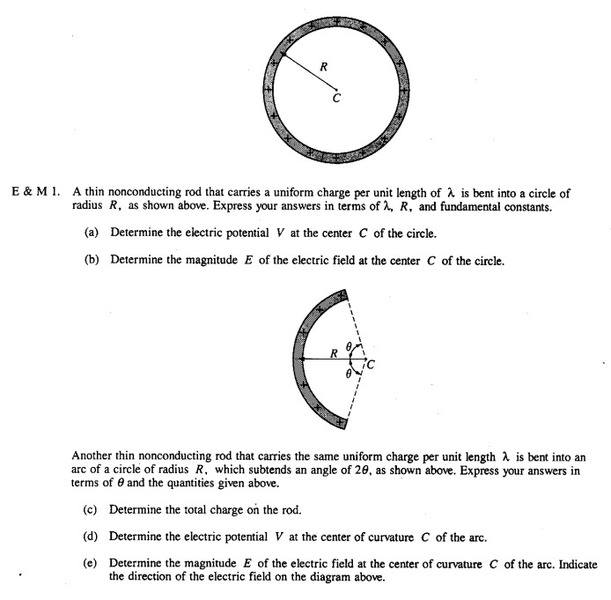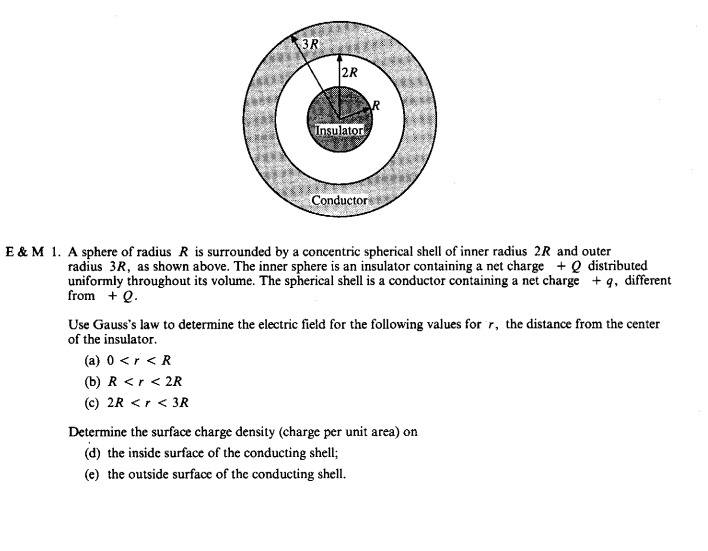# Need help with gauss law and charged ring AP Physics FRQ

## Homework Statement

1.2.## Homework Equations

∫E.dA = Qenc/$\epsilon$$_{o}$
$\lambda$=Q/L
$\rho$=Q/V
V= q/4πε$_{o}$r

## The Attempt at a Solution

these types of problems i suck at, i dont know how to do these at all independently, and is there any difference in question 2 if there is an insulator or not? is that irrelevant?

I have the scoring guidelines with the answers but they dont really teach anything

rude man
Homework Helper
Gold Member
, and is there any difference in question 2 if there is an insulator or not? is that irrelevant?

This is a simple exercise in applying Gauss's theorem.

To answer your question, yes it matters if the inside sphere is an insulator or a conductor. It matters for r < R.